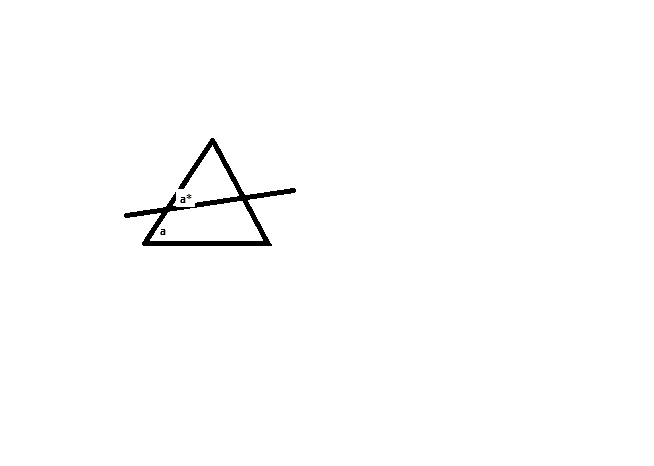# Trying to prove two angles are congruen to each other

cse63146

## Homework Statement

[PLAIN]http://img831.imageshack.us/img831/2589/88722757.jpg [Broken]

Using only the fact that the sum of the interior angles of a triangle is 180, how would I show that a = a* and b = b*?

## The Attempt at a Solution

In my diagram, there are 2 triangles: ABC and A*B*C

a + b + c = 180 and a* + b* + c =180 (a,b,a*,b,c are angles)

since both equations equal to 180, I equate them to each other and subtract angle c from both sides to get:

a + b = a* + b*.

I'm stuck here. I proved that their sum is the same, but not the angles. Do you have any suggestions?

Last edited by a moderator:

Homework Helper
hi cse63146!Using only the fact that the sum of the interior angles of a triangle is 180, how would I show that a = a* and b = b*?

well, they're only equal if the two lines are parallelare they parallel?

and if so, what consequences of being parallel are you allowed to use?cse63146
I'm trying to use the fact that a + b + c = 180, and somehow prove that ab and a*b* are parallel. If I can show that the two angles are congruent to another, then the lines would be parallel.

Mentor
What we need is the exact wording of the problem. It's not crystal clear what you are given to work with, and what you're trying to prove.

If all you have is the picture and the fact that the interior angles of a triangle add up to 180 deg., you don't have enough information to prove either that the lines are parallel or that a and a* and b and b* are congruent.

cse63146
The problem is:

Using the fact that the interior angles of a triangle sum up to 180, prove parallel transport (or PT!).

Homework Helper
Mentor
I have no idea what that (parallel transport) means.

Here's the problem. In this figure, angles a and a* are as in your drawing, and b and b* (not shown in my drawing) are in the same places.

The line through the middle of the triangle is supposed to obviously be not parallel to the base of the larger triangle. Even so, a + b + c = 180 deg., and a* + b* + c = 180 deg., so a + b = a* + b*, but that doesn't tell you anything about the individual angles.EDIT: I didn't realize my drawing would be so small. Hopefully you get the idea I'm trying to convey, anyway.

cse63146
Maybe this would help. The first part is to use Parallel Transport to prove that the sum of the interior angles sum up to 180.

Now I have to do the opposite.

#### Attachments

• PT.doc
106.5 KB · Views: 130
Mentor
Maybe this would help. The first part is to use Parallel Transport to prove that the sum of the interior angles sum up to 180.

Now I have to do the opposite.
This whole parallel transport business needlessly complicates things, IMO. All it means is that you are going to move a copy of a line segment rigidly so that it remains parallel.

The first part of the problem boils down to this: If L || the triangle base, then a = a' and b = b'. This can be proved very quickly. If L || the triangle base, then a = the vertical angle across from a'. Since vertical angles are congruent, then a and a' are congruent. Same argument for b and b'.

The second part boils down to this: If a line L intersects the sides of a triangle (as in your drawing) so that a = a' and b = b', the L is || the triangle base. Since a and a' are corresponding angles for two lines cut by a transversal, then the two lines are parallel, hence L is parallel to the base.

cse63146
The second part boils down to this: If a line L intersects the sides of a triangle (as in your drawing) so that a = a' and b = b', the L is || the triangle base. Since a and a' are corresponding angles for two lines cut by a transversal, then the two lines are parallel, hence L is parallel to the base.

I was thinking of doing something like that, but the question says that I have to use the fact that the interior angle of a triagngle sum up to 180.

Maybe it would help if I move the a*b* line somewhere else, hopefully it'll work.

Homework Helper
hi cse63146!(just got up :zzz: …)
The problem is:

Using the fact that the interior angles of a triangle sum up to 180, prove parallel transport (or PT!).

so are the diagram, and the angles a b a* and b*, in any way mentioned in the question, or are they merely part of your attempt to answer?if they're not mentioned in the question, you should forget them, and simply examine the definition you've been given of parallel transport

(also, try it on a sphere, where the angles always add to more than 180°, to see why parallel transport doesn't work there)